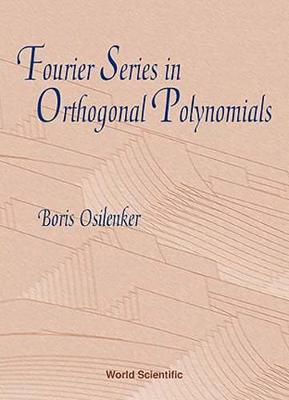•# Fourier Series In Orthogonal Polynomials (Hardback)

(author)
£69.00
Hardback 296 Pages / Published: 15/04/1999
• We can order this

Usually dispatched within 3 weeks

This book presents a systematic course on general orthogonal polynomials and Fourier series in orthogonal polynomials. It consists of six chapters. Chapter 1 deals in essence with standard results from the university course on the function theory of a real variable and on functional analysis. Chapter 2 contains the classical results about the orthogonal polynomials (some properties, classical Jacobi polynomials and the criteria of boundedness).The main subject of the book is Fourier series in general orthogonal polynomials. Chapters 3 and 4 are devoted to some results in this topic (classical results about convergence and summability of Fourier series in L2 ; summability almost everywhere by the Cesaro means and the Poisson-Abel method for Fourier polynomial series are the subject of Chapters 4 and 5).The last chapter contains some estimates regarding the generalized shift operator and the generalized product formula, associated with general orthogonal polynomials.The starting point of the technique in Chapters 4 and 5 is the representations of bilinear and trilinear forms obtained by the author. The results obtained in these two chapters are new ones.Chapters 2 and 3 (and part of Chapter 1) will be useful to postgraduate students, and one can choose them for treatment.This book is intended for researchers (mathematicians, mechanicians and physicists) whose work involves function theory, functional analysis, harmonic analysis and approximation theory.

Publisher: World Scientific Publishing Co Pte Ltd
ISBN: 9789810237875
Number of pages: 296
Weight: 540 g
Dimensions: 230 x 152 x 23 mm